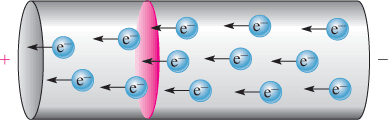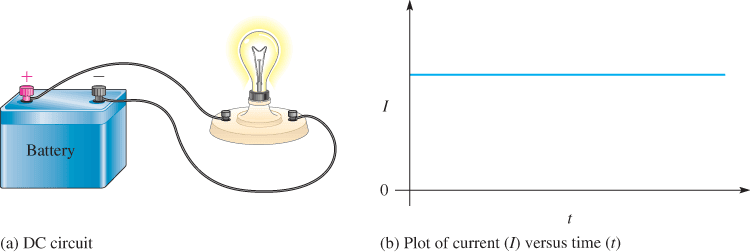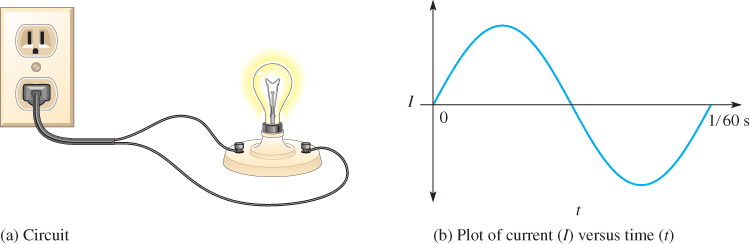# Electrical Current: Definition & Formula

Want create site? Find Free WordPress Themes and plugins.

The original idea of current was based on Benjamin Franklin’s belief that electricity was an unseen substance that moved from positive to negative. Conventional current is defined based on this original assumption of positive to negative.

The original definition is widely used, although there is another definition of current called electron flow. Electrons move from the negative to the positive point through the wires and components in a circuit, just opposite to the defined direction of conventional current. Some people prefer to think in terms of conventional current, while others prefer the electron flow definition.

## Definition of Current

Current is the flow of electrical charge past a specified point in a circuit. The word current implies a flow, so it is redundant to say “current flow.” Current is symbolizedFigure 1 Current.

Current is defined as the charge per time passing a point. If one coulomb of electrons passes through the cross-sectional area in 1 second, the current is 1 ampere.

by I and its unit is the ampere (A). One ampere of current is defined as a rate of charge flow equal to one coulomb per second, as illustrated in Figure 1. This is equivalent to 6.25 × 1018 electrons passing a point in one second, an enormous number of electrons. The power of ten, 1018, means that the decimal point in the number is moved 18 places to the right.

## The basic formula for current is

$I=Q/t$

Where

I = current, in amperes

Q = charge, in coulombs

t = time, in seconds

This equation is rarely applied to actual circuit work because the quantity of charge is generally not as important as the current itself. For practical reasons, the rate at which a charge moves past a point is defined, rather than the quantity of charge.

Direct Current

Current that is uniform in one direction is called direct current (dc). A dc voltage source such as a battery causes a direct current in a circuit. To have current in a circuit, the circuit must be complete, which means it has a voltage source, a load, and a closed path between the voltage source terminals. The load is a component such as a lamp or a heater that uses the power provided by the source. A simple complete dc circuit is shown in Figure 2(a), with a battery as the voltage source and a lightbulb for the load. The current in this circuit is steady, as illustrated in Figure 2(b).

Because the conductors connecting the battery to the lamp are solid wires, electrons comprise the moving charge in the circuit itself, but ions (charged particles) move in the fluid of the battery. The flow of electrons is from the negative terminal of the battery, through the load, and back to the positive terminal of the battery, where they complete a chemical reaction by combining with positive ions.Figure 2 Complete DC Circuit. The current is dc because the voltage source is a battery.Figure 3 In a circuit with an AC voltage source, the current reverses direction during one cycle.

Alternating Current

As mentioned earlier, electric utility companies produce sinusoidal ac voltage. When the ac voltage from the utility company is connected to a source to complete an electric circuit, a sinusoidal current with the same frequency as the voltage results. Figure 3 shows a circuit where the lamp is connected to the utility voltage from a wall outlet. The alternating current (ac) through the wires and lamp reverses direction during each cycle of the ac voltage, as indicated.

## Comparison of AC and DC

You might wonder how a steady current (dc) and a cyclic current (ac) can be compared. In ac, the charge reverses direction many times in a second, so current is constantly changing. The fact that it moves in one direction and then the other averages to zero, yet energy is still delivered to the load.

To make sense of ac and compare it to dc, engineers devised a measurement of current and voltage based on the equivalent electrical power that is delivered. If the ac is specified as root-mean-square (RMS) current and root-mean-square (RMS) voltage, the result is equivalent to a steady direct current and the direct voltage of the same values. This is the way ac is normally specified (unless stated otherwise). For example, 1 A of ac (RMS) is equivalent to 1 A of dc and 1 V ac (RMS) is equivalent to 1 V dc. So ac and dc can be compared directly when the ac is stated as an RMS value (the usual case).

Review

1. What is current, and what is its unit?
2. If an AC voltage source with a frequency of 60 Hz is connected to a circuit, what is the frequency of the current?